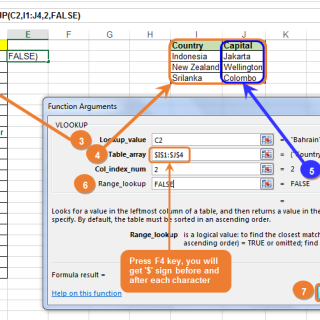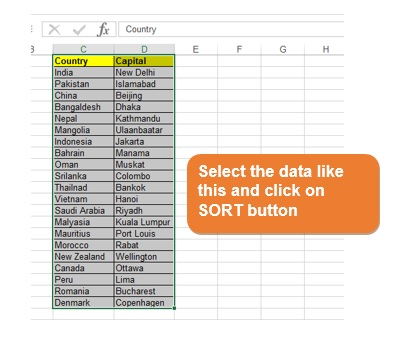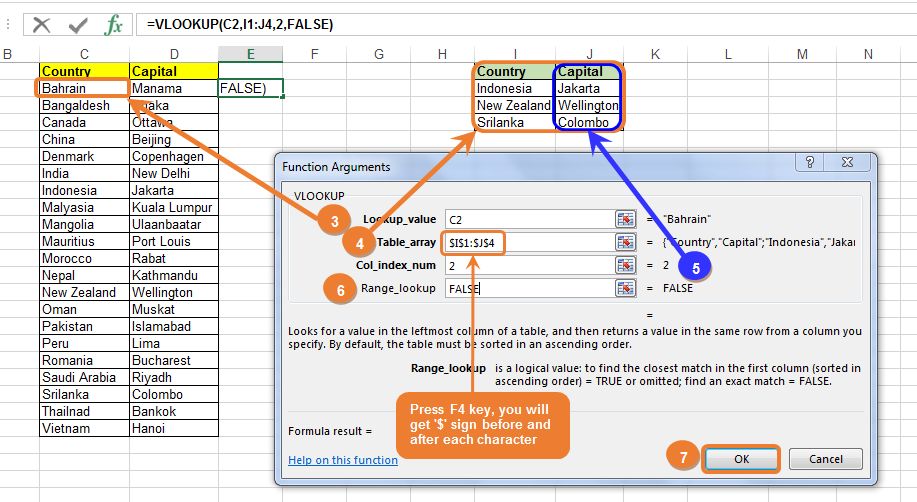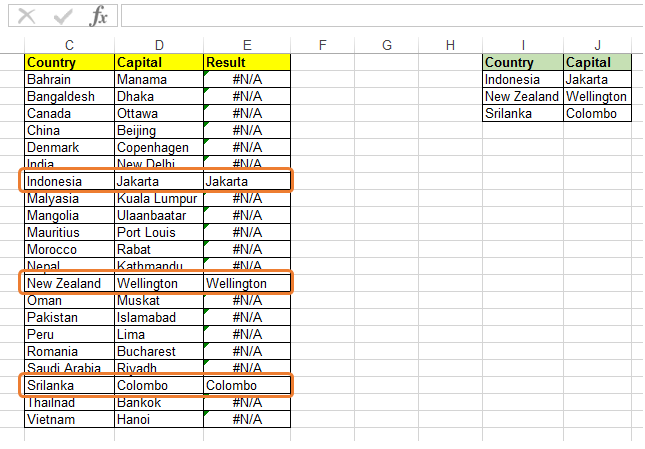# VLOOKUP in MS excel [in 4 Steps]

VLOOKUP in MS Excel:

VLOOKUP in MS excel is an important time saving function, which is helpful to every SAP Functional Consultant in various ways. Many times functional consultants come across situations where they have to search data in a certain column in ms excel sheet and match the data with another data base. Data set could be master data such as material master, customer master or transaction data such as Purchase Order, Sales Order data from respective tables or else dump from MSEG/ MKPF tables.

The secret to VLOOKUP is to organize your data so that the value you look up is to the left of the return value you want to find

Let us learn and apply VLOOKUP and make our work easier.

First we will understand the syntax of VLOOKUP formula.lookup_value : The data you want to look up or search. The data you want to look up must be in the first column of the range of cells you specify in table-array .

table_array : The range of cells in which the VLOOKUP will search for the lookup_value and the return value. The first column in the cell range must contain the lookup_value (for example, country in picture below.) The cell range also needs to include the return value (for example, Capital in the graphic below) you want to find.

col_index_num : The column number (starting with 1 for the left-most column of table-array) that contains the return value. In the below example if we enter column num as ‘2’, then it will return value of Capital.

range_lookup : A logical value that indicates whether we want VLOOKUP to search an exact match or an approximate match:

• TRUE assumes the first column in the table is sorted either numerically or alphabetically, and will then search for the closest value. This is the default method if you don’t specify one.
• FALSE searches for the exact value in the first column. We can also use ‘0’ (Zero) instead of FALSE. While working on SAP related work we use it zero.

Step 1: Below screen shows the Main Data set on left side and the value which we want to search is on left side.Step 2: First this that we must do before applying the formula is sorting of data in both tables.Click on SORT button a shown in the screenSORT second table in the same way.

Once sorting is done, data will look like this.Step 3 : Now we will apply the VLOOKUP formula. Check the below screen shots in sequence1. Click on VLOOKUP function, as shown in figure

2. Click on OK button.

Once you click OK a new window will pop up, then you will have to select the necessary data as shown in below figure.Meaning of each term in the above figure is already explained earlier. You can refer same. Go thru this diagram carefully and maintain entries in the given sequence.

3. Select the value which you want to search in table array.

4. The orange box is the table array (column I and J). Press F4 key to get \$ sign before and after each character. This will allow the formula to search correct value. Use of F4 key is best practice.

5. Here you can enter the column number which contains the return value. It is highlighted in blue color.

6. Here you can enter FALSE or ‘0’. If you enter TRUE here then this formula will search a closet value, not the exact value.

7. Finally click OK button.

Step 4: Now we will get the result in the cell number E2. Copy the formula in all the cells of column E. You will get the result for all records. Highlighted fields in below screen shot shows the result values.Errors in VLOOKUP and their meaning:

#N/A in cell : If range_lookup is TRUE, then if the value in the lookup_value is smaller than the smallest value in the first column of the table_array, you’ll get the #N/A error value. If range_lookup is FALSE, the #N/A error value indicates that the exact number isn’t found.

#REF! in cell : If col_index_num is greater than the number of columns in table-array, you’ll get the #REF! error value.

#VALUE! in cell : If the table_array is less than 1, you’ll get the #VALUE! error value.

#NAME? in cell : The #NAME? error value usually means that the formula is missing quotes. To look up a person’s name, make sure you use quotes around the name in the formula. For example, enter the name as “Fontana” in =VLOOKUP(“Fontana”,B2:E7,2,FALSE).

That’s all about VLOOKUP. I hope that this much of information is enough for an SAP consultant to understand the functionality of this formula.

### 1 Comment

•AJIT SAMBHUS February 7, 2017

Was looking for this with clean example,
VERY USEFUL & a MUST DOCUMENT,during implementation,
THANKS